Here we are providing Class 11 Physics Important Extra Questions and Answers Chapter 5 Laws of Motion. Important Questions for Class 11 Physics with Answers are the best resource for students which helps in Class 11 board exams.

## Class 11 Physics Chapter 5 Important Extra Questions Laws of Motion

### Laws of Motion Important Extra Questions Very Short Answer Type

Question 1.
(a) Why do we beat dusty blankets with a stick to remove dust particles?
It is done due to inertia of rest.

(b) A stone when thrown on a glass window smashes the window pane to pieces. But a bullet fired from a gun passes through it making a hole. Why?
This is due to the inertia of rest.

Question 2.
(a) If you jerk a piece of paper from under a book quick enough, the book will not move, why?
It is due to the inertia of rest.

(b) Passengers sitting or standing in a moving bus fall in forward direction when the bus suddenly stops. Why?
This is due to the inertia of motion.

Question 3.
(a) Why passengers are thrown outward when a bus in which they are travelling suddenly takes a turn around a circular road?
This is due to the inertia of direction.

(b) Is any force required to move a body with constant velocity?
No.

Question 4.
(a) Why a one rupee coin placed on a revolving table flies off tangentially?
This is due to the inertia of direction.

(b) Why mud flies off tangentially to the wheel of a cycle?
This is due to the inertia of direction.

Question 5.
(a) When the electric current is switched off, why the blades of a fan keep on moving for some time?
This is due to the inertia of motion.

(b) Why the passengers fall backward when a bus starts moving suddenly?
This is due to the inertia of rest.

Question 6.
(a) A body of mass m is moving on a horizontal table with constant velocity. What is the force on the table?
mg i.e. equal to the weight of the body.

(b) Name a factor on which the inertia of a body depends.
Mass.

Question 7.
(a) Rocket works on which principle of conservation?
Law of conservation of linear momentum.

(b) Is the relation $$\overrightarrow{\mathbf{F}}=\mathbf{m} \overrightarrow{\mathbf{a}}$$ applicable to the motion of a rocket?
No.

Question 8.
(a) Will a person while firing a bullet from a gun experience a backward jerk? Why?
Yes, it is due to the law of conservation of linear momentum.

(b) A bomb explodes in mid-air into two equal fragments. What is the relation between the directions of their motion? Answer:
The two fragments will fly off in two opposite directions.

Question 9.
(a) What happens to the acceleration of an object if the net force on it is doubled?
As a = $$\frac{F}{m}$$ i.e. a ∝ F, so acceleration will be doubled when m the force is doubled.

(b) An electron moving with a certain velocity collides against a stationary proton and sticks to it. Is the law of conservation of linear momentum true in this case?
Yes, it is true.

Question 10.
(a) According to Newton’s third law of motion, every force is accompanied by an equal (in magnitude) and opposite (in direction) force called reaction, then how can a movement take place?
As the action and reaction never act on the same body, so the motion is possible.

(b) You can move a brick easily by pushing it with your foot on a smooth floor, but, if you kick it, then your foot is hurt. Why?
As Ft remains constant, so if t is reduced, then F will be increased and hence hurt our foot.

Question 11.
(a) Why does a swimmer push the water backward?
So as to get forward push according to Newton’s third law of motion.

(b) Why does not a heavy gun kick so strongly as a light gun using the same bullets (i.e. cartridges)?
The recoil speed of the gun is inversely proportional to its mass. So the recoil speed of the heavy gun is lesser than that of the light gun.

Question 12.
(a) Can a rocket operate in free space?
Yes.

(b) In a game of tug of war, two opposing teams are pulling the rope with equal (in magnitude) but the opposite force of 1000 kg wt at each end of the rope. What is the tension in the rope if a condition of equilibrium exists?
1000 kg wt.

Question 13.
(a) Which of Newton’s laws of motion is involved in rocket propulsion?
Newton’s third law of motion.

(b) Action and reaction are equal in magnitude and opposite in direction, then why do not they cancel/balance each other?
They don’t balance each other as they act on different bodies.

Question 14.
(a) A passenger sitting in a bus at rest pushes it from within. Will it move? Why?
No, internal forces are unable to produce motion in a system.

(b) How would you explain the motion of a motorcyclist in a globe of death in a circus?
It is a case of motion in a vertical circle.

Question 15.
Can a body in linear motion be in equilibrium? why?
Yes, it will be in equilibrium if the vector sum of the forces acting upon the body is zero.

Question 16.
Is the law of conservation of momentum valid for a system consisting of more than two particles?
Yes, the law of conservation of momentum is a general law that is applicable to all systems.

Question 17.
Which is greater out of the following
(i) The attraction of 1 kg lead for Earth,
(ii) the attraction of Earth for 1 kg of lead? Why?
Both are equal, the forces of action and reaction are always equal and opposite in direction according to Newton’s third law of motion.

Question 18.
Mention the conditions for the maximum and minimum pull of a lift on a supporting cable.

1. The pull of the cable is minimum (zero) when the lift is falling freely.
2. The pull of the cable is maximum when the lift is moving up with the same acceleration.

Question 19.
A man is at rest in the middle of a pond on perfectly frictionless ice. How can he get himself to the shore of the pond?
He can get himself to the shore if he throws away his shirt or anything in his possession in a direction opposite to the desired direction of motion or by spitting in the forward direction or by blowing air from his mouth.

Question 20.
Suppose you are seated in a cabin that has no doors, no windows, etc., and is also soundproof. Shall it be possible to detect the uniform velocity with which this cabin is moving? Why?
No, this is because when the cabin is. moving with uniform velocity, there will be no net unbalanced force.

Question 21.
(a) Why do we pull the rope downwards for climbing up?
When we pull the rope downwards, an upward reaction helps us to rise up.

(b) Why is it easier to roll than to pull a barrel along a road?
It is due to the fact that rolling friction is less than sliding friction.

Question 22.
(a) Why are the lubricants used in machines?
Lubricants are used in machines so as to reduce friction.

(b) Friction is independent of the area, but brakes of a very small contact area are not used. Why?
This is done so as to avoid wear and tear.

Question 23.
Mention a factor on which coefficient of friction depends.
The coefficient of friction depends upon the nature of the surfaces in contact.

Question 24.
Carts with rubber tires are easier to ply than those with iron tires. Why?
The coefficient of friction between the rubber tires and the road is lesser than the coefficient of friction between iron and steel.

Question 25.
Why are wheels made circular? Explain.
Circular wheels roll without sliding on the road. Since rolling friction is less than sliding friction, so they move easily.

Question 26.
(a) What do you mean by dry friction?
When both the bodies in contact are solids, then the force of friction is called dry friction.

(b) A soda water bottle is falling freely. Will the bubbles of the gas rise in the water of the bottle?
A freely falling soda water bottle is in a state of weightlessness. Thus the bubbles of the gas will not rise in the water of the bottle rather they remain floating.

Question 27.
What do you mean by liquid or fluid or wet friction?
It is defined as the friction which conies into play between a surface and a liquid or fluid.

Question 28.
“Friction is a self-adjusting force.” Is this statement correct?
This statement is correct only so long as the friction is static friction. Up to the limiting friction, the force of friction is equal (in magnitude) and opposite to the applied force.

Question 29.
(a) Several forces act simultaneously on a body. In which direction will it move?
It will move in the direction of the net force.

(b) Name the physical quantity which is a measure of inertia of a body?
Inertial mass.

Question 30.
Can a force change only the direction of the velocity of an object keeping its magnitude constant?
Yes, a force can only change the direction of the velocity of an object keeping its magnitude constant.

Question 31.
(a) Two objects having different masses have some momentum. Which one of them will move faster?
The object with a smaller mass will move faster.

(b) A table is lying on the floor of a room. Is some force of friction acting on it?
No.

Question 32.
(a) A book is lying on an inclined plane. Is some force of friction acting on the book?
Yes

(b) Name the physical quantity which can be found from the area under the force-time graph.
Impulse

(c) Will the body be in equilibrium under the action of three non-coplanar forces.
No.

Question 33.
(a) Write the S.I. units of force, momentum, and impulse.
S.I. unis of force, momentum, and impulse are newton (N), kg ms-1, and Ns (Newton-second).

(b) Why should the hammer be heavier to push the nail deeper into the wooden blocks?
It should be heavier so as to increase the impact of force i.e. more force applied for a shorter time.

Question 34.
(a) Why rockets are given conical shapes?
The rockets are given conical shapes so as to reduce atmospheric friction.

(b) How does air friction affect the maximum height of a projectile?
The maximum height of a projectile is reduced due to air friction.

Question 35.
(a) Explain why jet planes cannot move in air-free space but rockets can move?
Jet planes use atmospheric oxygen to foil their fuel but rockets carry their own fuel and don’t depend on atmospheric oxygen.

(b) Is it correct to state that a body always moves in the direction of the net force acting on it?
The statement is true only for bodies at rest before the application of force.

Question 36.
At which place on Earth, the centripetal force is maximum?
The centripetal force is maximum at the equator.

Question 37.
What provides the centripetal force in the following cases?
(i) Electron revolving around the nucleus.
It is provided by
The electrostatic force of attraction between the electron and the nucleus.

(ii) Earth revolving around the sun.
The gravitational force of attraction between Earth and Sun.

(iii) Car taking a turn on a banked road.
A component of the reaction of the road.

Question 38.
(a) What is the direction of the angular velocity of the minute hand of a wall-clock?
The direction of the angular velocity of the minute hand of a wall- clock is perpendicular to the wall and directed inwards.

(b) What is the difference between ‘Newton’ and ‘newton’?
Newton is the name of a scientist but newton is the S.l. unit of force named after the scientist Newton.

Question 39.
(a) Is it possible that a particle moving with a constant velocity may not have a constant speed?
No.

(b) For uniform circular motion, does the direction of centripetal force depend on the sense of rotation {i.e. clockwise or anti-clockwise)?
No. It is always radial irrespective of the sense of rotation.

Question 40.
Why chinaware crockery is wrapped in paper or straws?
Paper or straw provides a cushion between th° pieces of crockery.
In case of any jerk (impulse), these will prolong the time of impact and reduce its effect, the crockery will thus be saved.

Question 41.
AH, vehicles are provided with springs and shockers, why?
To reduce the impact of force on the vehicle as shockers and springs break the impact of force and increase the time of action of force thus reduce the impulse and save the vehicle from shock.

Question 42.
A body falls from a single-story building roof on a muddy floor and another boy falls from the same height on a stone, who is likely to survive out of them and why?
The boy who falls on a muddy floor will survive due to the reduction of the impact of force by prolonging the time of reduction of force from maximum to zero.

Question 43.
What is the effect on the direction of centripetal force when the revolving body reverses its direction of motion?
The centripetal force will be directed towards the center of the circle. This fact does not depend upon the sense of the rotation of the circle.

Question 44.
Is it correct to say that the banking of roads reduces the wear and tear of the tires of automobiles? If yes explain.
Yes, if the road is not banked, then the necessary centripetal force will be provided by the force of friction between tires and the road. On the other hand; when the road is banked, a component of the normal reaction provides the necessary centripetal force, which reduces wear and tear.

Question 45.
The linear velocity of a particle moving on the circumference of the circle is equal to the velocity acquired by a freely falling body through a distance equal to one-fourth the diameter of the circle. What is the centripetal acceleration of the particle moving along the circle?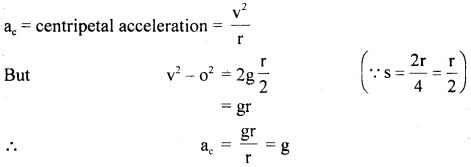Question 46.
(a) A body is moving with uniform velocity. Can it be said to be in equilibrium? Why?
Yes, it can be said to be in equilibrium when it moves with uniform velocity as no acceleration i.e. no net force acts on the body.

(b) Why Newton’s second law of motion is not applicable to the motion of a rocket?
Newton’s second law i.e. F = ma is applicable only if the mass (m) of the body remains constant. In the case of the rocket, the mass continuously decreases and hence F = ma is not applicable.

Question 47.
(a) A thief jumps from the upper story of a house with a load on his back. What is the force of the load on his back when the thief is in the air?
When the thief is in the air, he is in the state of free fall, and hence in the state of weightlessness. So the force of the load on his back is zero.

(b) When a body falls to the Earth, the Earth also moves up to meet it. But the motion of Earth is not noticeable. Why? Answer:
We know that acceleration is the ratio of the applied force to the mass of the body. As the mass of Earth is very large, so its acceleration is very small.

Question 48.
What are the different effects a force is capable of producing?
Force can cause the following

1. Circular motion
2. Translatory motion
3. Deformations in the body on which it is applied.

Question 49.
A ball is thrown up at a speed of 36 ms-1 by a thrower. If the ball returns to the thrower with the same speed. Will, there be any change in:
(a) Momentum of the ball?
There will be a change in the direction of the momentum of the ball.

(b) Magnitude of the momentum of the ball?
There is no change in the magnitude of the momentum of the ball.

Question 50.
When a ball falls from a height its momentum increases. What causes the increase in the momentum of the ball?
The gravitational force acting in the direction of motion increases the velocity and hence momentum of the ball.

Question 51.
When a high jumper leaves the ground, where does the force which accelerates the jumper upward comes from?
The high jumper after taking a short run presses the ground hard, the ground, in turn, reacts on him and provides the necessary upward accelerating force to the jumper. Thus, the reaction of the ground on the jumper is the required force.

Question 52.
Name the forces which are in equilibrium in each of the following situations:
(a) a book resting on a table.
The gravitational force on the book and a force of reaction of the table.

(b) a cork floating on water.
The gravitational force on the cork and an upward thrust or buoyant force of water.

(c) a pendulum bob suspended from the ceiling with the help of a string.
The gravitational force on the bob and the tension in the string.

### Laws of Motion Important Extra Questions Short Answer Type

Question 1.
(a) A learner shooter fired a shot from his rifle and his shoulder got injured ¡n the process. What mistake did he commit?
We know that a gun recoils i.e. moves back after firing. To avoid injury to the shoulder, the gun must he held tightly against the shoulder. The learner shooter might have not held it tightly against his shoulder and hence the gun must have injured his shoulder after firing.

(b) When the horse suddenly stops, the rider falls in the forward direction. Why? Explain it.
When the horse suddenly stops, the rider falls in forwarding direction due to the inertia of motion.

Explanation: The lower portion of the rider comes to rest along with the horse while the upper portion of the rider continues to move forward. Hence, he falls forward.

Question 2.
(a) Newton’s first law of motion is the law of Inertia. Explain.
According to Newton’s first law of motion, a body can’t change its state of rest or of uniform motion along a straight line unless an external force acts on it. It means that the natural tendency of the material body is to continue in the state of rest or that of uniform motion which is termed as inertia. Thus Newton’s first law is the law of inertia.

(b) What happens to a stone tied to the end of a string and whirled in a circle if the string suddenly breaks? Explain why?
The stoneflies off tangentially to the circle along a straight line at the point where the string breaks. It is due to the inertia of direction. When the string breaks, the force acting on the stone ceases. In the absence of force, the stoneflies away in the direction of instantaneous velocity which is along the tangent to the circular path.

Question 3.
(a) An astronaut accidentally gets separated out of his small spaceship accelerating in inter-steller space at a constant rate of 100 ms-2. What is the acceleration of the astronaut at the instant after he is outside the spaceship?
According to Newton’s first law of motion, the moment he is out of the spaceship, there is no external force on the astronaut, thus his acceleration is zero. Here we are assuming that he is out of the gravitational field of heavenly bodies i.e. there are no nearby stars to exert a gravitational force on him and the small spaceship exerts a negligible gravitational attraction on him.

(b) How is it that a stone dropped from a certain height falls much more rapidly as compared to a parachute under similar conditions?
As the surface area of a parachute is much larger as compared to the surface area of a stone, so the air resistance, i. e. fluid friction in the case of the parachute is much larger than in the case of stone. Hence the parachute falls slowly.

Question 4.
(a) When a man jumps out of a boat, then it is pushed away. Why?
This is due to Newton’s third law of motion. When the man jumps out of the boat, he applies a force on it in the backward direction, and in turn, the reaction of the boat on the man pushes him out of the boat.

(b) Explain how lubricants reduce friction?
The lubricants spread as a thin layer between the two surfaces. The motion now is between the surface and the lubricant layer which changes the dry friction into wet friction. As wet friction is less than dry friction, hence lubricants reduce friction.

Question 5.
Two hoys on ice-skates hold a rope between them. One boy is much heavier than the other. The lightweight boy pulls on the rope. How will they move?
The light-weight boy is doing the action on the heavy boy by pulling the rope. According to Newton’s third law, equal and opposite force (reaction) also acts on the light boy. As the mass of the boy pulling the rope is lesser, so the acceleration produced in him will be more. Thus both the boys move tow; rds each other and the lighter boy will move faster.

Question 6.
Explain why ball bearings are used in machinery?
We know that rolling friction is much lesser than sliding friction, so we convert the sliding friction into rolling friction which is done using ball bearings that are placed in between the axle and the hub of the wheel. The ball bearings tend to roll around the axle as the wheel turns and as such the frictional force is reduced.

Question 7.
Why a horse has to apply more force to start a cart than to keep it moving? Explain.
Static friction comes into play when the horse applies force to start the motion in the cart. On the other hand, kinetic friction comes into play when the cart is moving.

Also, we know that the static friction is greater than the kinetic friction, so the horse has to apply more force to start a cart than to keep it moving.

Question 8.
Sand is thrown on tracks or roads covered with snow. Explain why?
When the roads (or tracks) are covered with snow, then there is a considerable reduction of frictional force between the tires of the vehicles and the road (or between the track and the wheels of the vehicle or train) which leads to the skidding of the vehicles (or trains). Thus, driving is not safe. When sand is thrown on the snow-covered roads (or tracks), then the force of friction increases, so safe driving is possible.

Question 9.
Explain why is it difficult to move a cycle along a road with its brakes on?
When we move the cycle with its brakes on, then its wheels can only skid i.e. slide along the road as they can’t rotate. So the friction is sliding in nature. As the sliding friction is greater than fr,e rolling friction, therefore, it is difficult to move a cycle with its brakes on.

Question 10.
Explain how proper inflation of tires saves fuel?
When the tires are properly inflated, the area of contact between the tires and the ground is reduced which in turn reduces the rolling friction. As a result of this, there is less dissipation of energy against friction. So the cover of the automobile/eater distance for the same quantity of fuel consumed. Hence proper inflation of tires leads to saving fuel.

Question 11.
Explain how the man at rest in the middle of a pond of perfectly frictionless ice comes out by blowing air or splitting etc.?
By blowing air from b’s mouth in a forwarding direction, the man applies some force in that direction and hence imparts some momentum to the air blown out from his mouth. According to the law of conservation of linear momentum, an equal and opposite momentum will be imparted to his body. Since there is no force of friction and hence no energy is wasted. Finally, by doing the same action again and again he gets himself on the shore.

Question 12.
Give one argument in favor of the fact that frictional force is a non-conservative force.
The direction of the frictional force is opposite to the direction of motion. When a body is moved, say from point A to B and then back to A, then work is required to be done both during forward and backward motion. So the network done in a round trip is not zero. Hence the frictional force is a non-conservative force.

Question 13.
Why is it more dangerous to fall on frozen ice than on fresh snow?
The fresh snow is softer and frozen ice is harder. So the impulse caused by falling on fresh snow is much lesser than frozen snow. Thus, falling on frozen ice may cause serious injury or maybe fatal (the reaction will be much greater in this case).

Question 14.
(a) An astronaut in open space is away from his spaceship. How can he return to his ship?
Using Newton’s third law of motion, he can reach his spaceship. He must throw objects with appropriate force opposite to the direction of the spaceship. The recoil will send him-to his spaceship.

(b) Why mudguards are used in vehicles?
The mud stuck to the tires fly off tangentially to the tires due to the inertia of direction. The mudguards are i so placed that they come in the way of mud and it is stopped by them from spilling all over.

Question 15.
What is the difference between absolute and gravitational units of force?
The absolute units of force don’t depend upon the value of acceleration due to gravity, so they remain the same throughout the universe. But the gravitational units of force depend upon the value of ‘g’ which is different at different places.

Question 16.
A disc of mass m is placed on a table. A stiff spring is attached to it and is vertical. To the other end of the spring is attached a disc of negligible mass. What minimum force should be applied to the upper disc to press the spring such that the lower disc is lifted off the table when the external force is suddenly removed?
The minimum force should be mg. When a force mg is applied vertically downwards on the upper disc, the lower disc will be pressed against the floor with a force mg. The floor will exert an upward reaction mg. When the external force is suddenly removed, this reaction will just lift the lower disc.

Question 17.
A force acting on a material particle of mass m first grows to a maximum value Fm and then decreases to zero. The force varies with time according to a linear law, and the total time of motion is tm. What will be the velocity of the particle at the end of this time interval if the initial velocity is zero?
In the given problem,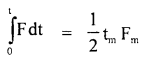= area under F – t graph
= area of ΔOAB ..(1)

Also by definition of impulse, we know that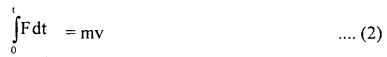Where m = mass of the particle,
v = its velocity after time interval
i.e. after time tm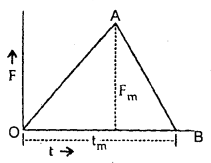∴ From (1) and (2), we get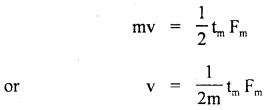Question 18.
Sometimes we need to increase friction. Why? Given an example.
Sometimes friction between two surfaces decreases to such an extent that it is difficult to move on that surface. So friction needs to be increased. For example, vehicles can’t move on a road covered with snow. In such cases, we have to throw sand on the road to increase the friction.

Question 19.
Vehicles stop applying brakes. Does this phenomenon violate the principle of conservation of momentum?
The law of conservation of momentum is not violated by stopping vehicles from applying brakes. Some retarding force is being applied due to brakes and the vehicle comes to rest such that the total loss of its momentum is equal to the impulse of the applied force. Thus the law of conservation of momentum is not violated.

Question 20.
“Two surfaces if made extremely smooth, will have a very low value of friction between them.” Is the statement true? Justify your answer with two illustrations.
The given statement is wrong. This is because the force of frictions increased when the surfaces in contact are made highly smooth.

Examples:

1. In factories, the conveyer belt is made smooth by rubbing it with wax or resin. The wheel over which the belt is to move is also made extremely smooth. Due to the increased force of friction between the belt and the wheel, slipping does not take place.
2. The wheels of the railway train and the surfaces of the railway tracks are also made very smooth.

Question 21.
Why force of friction increases when the two surfaces in contact are made extremely smooth i.e. polished beyond a certain limit?
When the two surfaces in contact are made extremely smooth, then they come in intimate contact with each other. So the force of adhesion comes into play- Due to this force, the motion of one surface over the other surface becomes retarding and hence causes an increase in the friction.

Question 22.
(a) A small amount of water spread on a marble floor causes slipping. Why?
Water fills the grooves in the floor and makes it more smooth thus reducing friction and it causes slipping.

(b) Why automobile tires have generally irregular projections over their surface?
The automobile tires have generally irregular projections over their surface so as to

1. Increase friction.
2. Increase the grip with the ground and thus avoiding their skidding.

Question 23.
A body moving over the surface of another body suddenly comes to rest. What happens to friction between the two surfaces?
The force of friction will be present only so long as there is relative motion between the two bodies and it automatically disappears as soon as the relative motion ceases.

Question 24.
Explain why one should take short steps rather than long steps while walking on ice?
There is a danger of slipping if one walks on ice by taking long steps. This can be explained as –

Explanation: Let R represents the reaction offered by ice while walking on it.
Also, let mg = weight of the person walking on the ice.

Let f = force of friction between ice and the foot of the person walking on it.
Let θ = angle made by R with the vertical.The rectangular components of R are shown in the figure. The vertical component i.e. R cos θ will balance the weight of the person and the horizontal component i.e. R sin θ will help the person to walk forward by balancing the f.
i. e. R cos θ = mg …(i)
and R sin θ = f …(ii)

Dividing (ii) by (i), we getwhere μ = coefficient of friction and has a fixed value.

If steps are longer, then θ is more, so tan θ will be more. But as p. is fixed, so f is to be increased which is not possible, hence there is a danger of slipping in a long step.

Question 25.
Why a cricket player lowers his hands while catching a cricket ball?
While taking a catch, a cricket player moves his hands backward. He has to apply retarding force to stop the moving ball in his hands. If he catches the ball abruptly, then he has to apply a large retarding force for a short time. So he gets hurt.

On the other hand, if he moves his hands backward then the player applies the force for a longer time to bring the ball to rest. In this case, he has to apply less retarding force and thus will not get hurt.

Thus to avoid injuries to his hands, he lowers his hands while catching a cricket ball.

Question 26.
Why are buffers provided between the bogies of a railway train?
Buffers are provided between the bogies of a train to reduce shocks or jerks by reducing the impulse or impact of a force. Springs of buffers increase the time of contact in the collision of bogies and thus the force acting on the bogies will be small and hence the passengers sitting inside the train will feel fewer jerks.

Question 27.
It is more difficult to catch a cricket ball than to catch a tennis ball moving with the same velocity. Explain why?
The mass of a cricket ball is greater than the mass of a tennis ball, so the change in momentum of the cricket ball is more than that of the tennis ball. Hence greater force is required to catch the cricket ball than that of the tennis ball.

Question 28.
(a) A body of mass 25 g is moving with a constant velocity of 5 ms’1 on a horizontal frictionless surface in a vacuum, what is the force acting on the body?
F = ma, where a = acceleration of the body.
For constant velocity, a = 0, so F = 0.
Thus when a body moves with constant velocity then no force acts on it.

(b) A bird is sitting on the floor of a wire cage and the cage is in the hand of a boy. The bird starts flying in the cage. Will the boy experience any change in the weight of the cage? Why?
Lighter. Since the cage is made of wire, the air inside the cage is not bound with the cage, rather it is in contact with the atmospheric air. Therefore the boy will not experience the weight of the bird when it flies in the cage. Thus the cage will appear lighter when the bird starts flying in the cage.

Question 29.
A woman stands on a spring scale on an elevator. In which case will the scale record the minimum reading and the maximum reading?
(i) elevator stationery,
When the elevator is stationary, then reading of the scale = actual weight of woman = mg.

(ii) elevator cable breaks, free fall,
When the elevator falls freely, it is the case of weightlessness.
∴ Reading of the scale = m(g – g) = 0 (∴ a = g)

(iii) elevator accelerating upward and
When the elevator accelerates upwards, the reading of the scale = m(g + a), where a = acceleration of the elevator.

(iv) elevator accelerating downward.
When the elevator accelerates downwards, then the reading of the scale = m(g – a).
Thus, the reading of the scale is minimum when the elevator falls freely and the reading of the scale is maximum when the elevator accelerates upwards.

Question 30.
Two bodies of different masses m1 and m2 are falling from the same height. If resistance offered by the air be the same for both the bodies, then will they reach the Earth simultaneously? Assume m1 > m2?
Let f be the resistance offered by the air for both the bodies.
If F = Net force acting on the body of mass m, i.e. weight W1 = m1g,Then F = W1 – f
= m1g – f …(1)

If a1 = acceleration produced in the body of mass m, then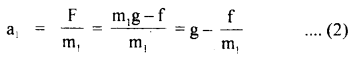Similarly if a2 be the acceleration produced in the body of mass m2,
then
a2 = g – $$\frac{\mathrm{f}}{\mathrm{m}_{2}}$$ ….(3)

Now as m1 > m2, so it is clear from equation (2) and (3) that
a2 < a1 or a1 > a2.

Thus, we conclude that the body having larger acceleration will reach earlier, so the body of larger mass will reach the earth earlier than the body of smaller mass.

Question 31.
How does air friction affect the maximum height of a projectile?
The air friction reduces both vertical and horizontal components of velocity. The air friction depends on the volume of the body or the air displaced. So the height attained by the body in projectile motion is severely restricted by the air friction. The height reached is reduced compared to one which is attained in the absence of air.

Question 32.
Why is it difficult to climb a greasy pole or rope?
For climbing any pole or rope, the climber presses the pole or rope with his feet which in turn pushes the feet and the person climbs up. But the man’s feet push the pole only if there is friction between the pole and the feet so that feet can hold on.

When grease is applied to the pole, the friction is sharply reduced and the foot cannot press it in the absence of friction. Thus, man does not get the reaction required to climb, hence it is difficult to climb a greasy pole or rope.

Question 33.
Does F = ma represent Newton’s second law of motion under all conditions? Give a reason for your answer.
No, F = ma does not represent Newton’s second law of motion under all conditions. In fact the statement of Newton’s second law of motion relates force with a change in momentum of the body i. e.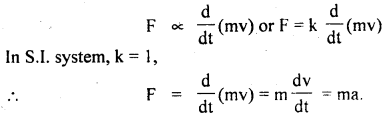In a reference frame where the velocity of the body is too high i.e., it approaches the speed of light then mass does not remain the same. Thus, in that case,holds goods which are different from F = ma.

Question 34.
Several passengers are standing in a running bus, it is said to be dangerous. How will you justify the statement?
There are three reasons for such situations to be dangerous –

1. Standing passengers raise the center of gravity of the bus and thus the bus comes in unstable equilibrium. This becomes dangerous.
2. A sudden brake applied by the driver will cause passengers to fall forward one over the other. Thus, they may be injured and there may be stamped.
3. If the bus is suddenly stopped, the passenger’s forward momentum may push it slightly ahead which may cause the accident in a congested traffic situation.

Question 35.
In a circus in the game of swing, the man falls on a net after leaving the swing but he is not injured, why?
When the man falls on the net it is depressed where a man falls on it and thus the time of contact is increased. Due to this, the force of reaction on the man is reduced to a great extent. Because the increase in time reduces the impulse which is equal to the changes in momentum (FΔt = m Δv). So, F is quite less and the man is not injured by the net. In fact, F pushes him up once and again he falls on the net.

Question 36.
(a) A meteorite burns in the atmosphere before it reaches the Earth’s surface. What happens to its momentum?
A meteorite while traveling towards earth shares its momentum with atmospheric particles and the remaining momentum is imparted to the Earth.

(b) By accident a person fell on a floating ice slab in a pond. He has nothing with him to get out and the ice slab is big enough so that he cannot put his hands or feet in the water. How can he save himself by coming out of the ice slab?
The person should know how to generate a force so that the ice slab is thrown away opposite to the direction of the force along with. This, he can do by spitting forcefully or sneezing, etc. again and again till the slab reaches the shore of the lake from where he can get out from the slab by the same technique.

Question 37.
In pushing a box up an inclined plane, is it better to push horizontally or to push parallel to the inclined plane? Why?
The box should be pushed by a force parallel to the plane. This will neither increase the reaction nor the force of friction. If the force is applied horizontally, only a component of the force will push the box up but the second component acting normally to the inclined plane will increase the reaction of the plane and thereby increase the force of friction. This will make the pushing of the box up the inclined harder.

Question 38.
Explain the role of friction in the case of bicycle brakes. What will happen if a few drops of oil are put on the rim?
When the brakes are applied, the rubber padding creates

1. a normal force on the rim, and the brakes and
2. force of friction are created in the direction opposite to the rotation of the wheel.

Thus, brakes stop the rotation of the wheels and the cycle is stopped.

If a few drops of oil are put on the rim, the friction is reduced but brakes apply a force on the rim which increases the normal reaction and thereby the force of friction. No doubt that now bicycle will take a longer time to stop and a small amount of oil will be thrown out due to centrifugal force.

Question 39.
A man cannot run faster on the sandy ground but a snake can. Why?
The greater friction of sandy ground stops the man from running faster but the snake gets support as its points of contact with the ground help in holding and pushing its body. The normal reaction of man is much more as compared to that of snake, that too is a factor in the relative force of friction acting on the two.

### Laws of Motion Important Extra Questions Long Answer Type

Question 1.
(a) State and prove impulse-momentum Theorem.
It states that the impulse of force on a body is equal to the change in momentum of the body.
i.e. J = Ft = P2 – P1

Proof: From Newton’s Second law of motion, we know that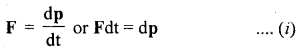Let P1 and P2 be the linear momenta of the body at time t = 0 and t respectively.
∴ integrating equation (i) within these limits, we get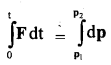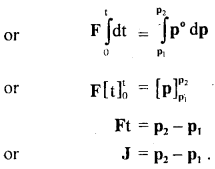Hence proved.

(b) Prove that Newton’s Second law is the real law of motion.
Proof: If we can show that Newton’s first and third laws are contained in the second law, then we can say that it is the real law of motion.
1. First law is contained in second law: According to Newton’s second law of motion,
F = ma …(i)
where m = mass of the body on which an external force F is applied and a = acceleration produced in it.

If F = 0, then from equation (1), we get
ma = 0, but as m ≠ 0
∴ a = 0

which means that there will be no acceleration in the body if no external force is applied. This shows that a body at rest will remain at rest and a body in uniform motion will continue to move along the same straight line in the absence of an external force. This is the statement of Newton’s first law of motion. Hence, the First law of motion is contained in the Second law of motion.

2. Third law is contained in second law: Consider an isolated system of two bodies A and B. Let them act and react internally.
Let FAB = force applied on body A by body B
and FBA = force applied on body B by body A

It $$\frac{\mathrm{d} \mathbf{p}_{\boldsymbol{A}}}{\mathrm{dt}}$$ = rate of change of momentum of body A
and
$$\frac{\mathrm{d} \mathbf{p}_{\boldsymbol{B}}}{\mathrm{dt}}$$ = rate of change of momentum of body B

Then according to Newton’s second law of motion,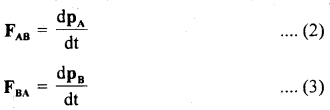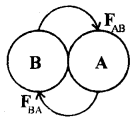(2) and (3) givesAs no external force acts on the system (∵ it is isolated), therefore according to Newton’s second law of motion,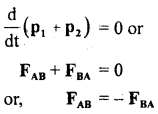or
Action = – Reaction,
which means that action and reaction are equal and opposite. It is the statement of Newton’s 3rd law of motion. Thus 3rd law is contained in the second law of motion.

As both First and Third Law is contained in Second law, so Second law is the real law of motion.

Question 2.
Derive the general expression for the velocity of a rocket in flight and obtain the expression for the thrust acting on it.
The working of a rocket is based upon the principle of conservation of momentum. Consider the flight of the rocket in outer space where no external forces act on it.
Let mo = initial mass of rocket with fuel.
Vu = initial velocity of the rocket,
m = mass of the rocket at any instant t.
v = velocity of the rocket at that instant.
dm = mass of the gases ejected by the rocket, in a small-time it.
u =H velocity of exhaust gases,
DV = increase in the velocity of the rocket in a time dt.

∴ Change in the momentum of exhaust gases = dm. u
Change in momentum of rocket = – (m – dm) dv.

A negative sign shows that the rocket is moving in a direction opposite to the motion of exhaust gases.

Applying the law of conservation of linear momentum,
dm.u = – (m – dm) dv …(1)

As dm being very small as compared to m, so it can be neglected, Thus, eqn. (1) reduces to
dm.u = – m dv
or
dv = – u $$\frac{dm}{m}$$ …(2)

Instantaneous velocity of the rocket:
At t = 0, mass of rocket = m0, velocity of rocket = vo.
At t = t, mass of rocket = m, velocity of rocket = v.

∴ Integrating Eqn. (1) within these limits, we get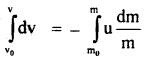In actual practice, the velocity of exhaust gases nearly remains constant.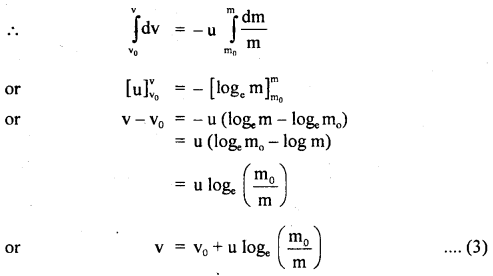equation (3) gives the instantaneous velocity of the rocket. In general vo = 0 at t = 0,

∴ Eqn. (3) reduces to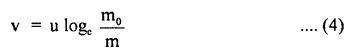From Eqn. (4), we conclude that the velocity of the rocket at any instant depends upon:

1. speed (u) of the exhaust gases.
2. Log of the ratio of initial mass (m0) of the rocket to its mass (m) at that instant of time.

Upthrust on the rocket (F): It is the upward force exerted on the rocket by the expulsion of exhaust gases. It is obtained as follows:
Dividing Eqn. (2) by dt, we get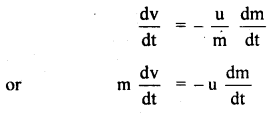where F = ma is the instantaneous force (thrust).

From Eqn. (5), we conclude that the thrust (F) on the rocket at any instant is the product of the velocity of exhaust gases and the rate of combustion of fuel at that instant. Here negative sign shows that the thrust and velocity of exhaust gases are in opposite direction.

Question 3.
(a) Define inertia. What are its different types? Give examples.
The tendency of bodies to remain in their state of rest or uniform motion along a straight line in the absence of an external force is called inertia.

Inertia is of the following three types:
1. The inertia of rest: When a body continues to lie at the same position with respect to its surrounding, it is said to possess inertia of rest. This situation may be changed only by the application of external force. For example, if a cot or sofa is lying in a particular place in the house, it will remain there even after days or years unless someone removes (by applying force) the same from its position. This is an example of the inertia of rest.

2. The inertia of motion: When a body is moved on a frictionless surface or a body is thrown in a vacuum, it will continue to move along its original path unless acted upon by an external force. In actual situations, air or floor etc. exert friction on the moving bodies so we are unable to visualize a force-free motion. This type of inertia when a body continues to move is called the inertia of direction.

3. In the above examples it is found that the direction of motion of the body or particle also does not change unless an external force acts on it. This tendency to preserve the direction of motion is called the inertia of direction.

(b) Explain Newton’s First law of motion. Why do we call it the law of inertia?
According to the First law of motion, “Everybody continues to be in the state of rest or of uniform motion along a straight line until it is acted upon by an external force.”

It means that if a book lying on a table,-it will remain there for days or years together unless force is applied on it from outside to pick it.

Similarly, if a body is moving along a straight line with some speed, it will continue to do so until some external force is applied to it to change its direction of motion.

Thus First law tells us the following:

1. It tells us about the tendency of bodies to remain in the state of rest or of motion and the bodies by themselves can neither change the state of rest nor of uniform motion. This tendency is called inertia. To break the inertia of rest or motion or direction, we need an external force. Thus the definition of the first law matches with the definition of inertia and hence first law is called the law of inertia.
2. The first law of motion also provides the definition of another important physical quantity called force. Thus force is that agency which changes or tends to change the state of rest or of uniform motion of a body along a straight line.

(c) State Newton’s Second law of motion. How does it help to measure force? Also, state the units of force.
It states that the time rate of change of momentum of a body is directly proportional to the force applied to it.where a = $$\frac{dv}{dt}$$ = acceleration produced in the body of mass m.
k = proportionality constant which depends on the system of units chosen to measure F, m, and a.

In the S.I. system, k = l,
∴ F = ma

The magnitude of the force is given by
F = ma …. (2)

Note: We have assumed that the magnitude of velocity is smaller and much less than the speed of light. Only under this condition Eqns. (1) and (2) hold good.

The definition of the Second law and its mathematical form is given in Eqn. (2) provide us a mean of measuring force.

One can easily find the change in velocity of a body in a certain interval of time. Both velocity and time can be easily measured. Thus by knowing the mass of the body one can determine both change in momentum as well as the acceleration of the body produced by an external force. If the force is increased, the rate of change of momentum is also found to increase. So also is the acceleration. Now with known values of m and we can find F.

Units of force: Force in S.I. units is measured in newton or N. From Eqn. (1) or (2) we can see that a newton of force is that fore? which produces 1 ms-2 acceleration in the body of mass 1 kg.
1 newton = 1 kilogram × 1 metre/(second)2
or
1 N = 1 kg × 1 ms-2 = 1 kg ms-2

In CGS system force is measured in dyne
1 dyne = 1 gram × 1 cm/s2 = 1 g cm s-2
Since 1 N = 1 kgm s-2= 1000 g × 100 cm s-2
= 105 g cm s-2 = 105 dyne
1 N = 105 dyne
or
1 dyne = 105 N

Gravitational Unit: If a falling mass of 1 kg is accelerated towards the Earth with 9.8 ms-2, then the force generated is called 1 kg wt (1-kilogram weight) force. It is the S.I. gravitational unit of force.

We know that the earth accelerates the mass with g = 9.8 ms-2
1 Kg wt = 9.8 N [1 kg × 9.8 ms 2 = 9.8 N]

C.G.S. gravitational unit is gf or g wt.
1 gf = 1g × 980 cms-2
= 980 dyne

Question 4.
(a) State Newton’s Third law of motion. Discuss its consequences.
Newton’s Third law of motion states that “to every action, there is always an equal and opposite reaction.”’
So, if a body 1 applies a force F12 on body 2 (action), then body 2 also applies a force F2] on body 1 but in opposite direction, then
F21 = – F12

In terms of magnitude
|F21| = |-F12|

It is very important to note that F12 and F21 though are equal in magnitude and opposite in direction yet act on different points or else no motion will be possible.

For example, hands pull up a chest expander (spring), and spring in turn exerts a force on the arms. A football when pressed reacts on the foot with the same force and so on. The most important consequence of the third law of motion is the law of conservation of linear momentum and its application in collision problems.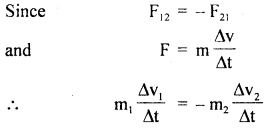Here Δt is the time for which the bodies come in contact during impact. This is the same for the two bodies of masses m1 and m2 and having velocity changes Δv1 and Δv2 respectively. Therefore,
m1 Δv1 = – m2 Δv2
or
m1 Δv1 + m2Δv2 = 0

Let u1, u2 and v1 and v2 be initial and final velocities of the two masses before and after collision, then
m1(v1 – u1) = – m2(v2 – u2)
or
m1u1 +m2u2 = m1v1 + m2v2

Momentum before impact = momentum after impact
This is the law of conservation of momentum.

(b) State the law of conservation of linear momentum and illustrate it with examples.
‘The linear momentum of an isolated system always remains the same provided no external force is applied on it.’ This is the law of conservation of linear momentum.

The linear momentum of a body = mass × velocity
p = mv
If a system has several bodies initially at rest then initial momentum = 0.
The final momentum = p1 + p2 + p3 + ……

According to law of conservation of linear momentum
p1 + p2 + p3 + …. = 0

Linear momentum is a vector quantity and is measured. in kg ms-1or Ns.

For example, a gun and a bullet make a system in which both are initially at rest. When the bullet of mass m is
fired with muzzle velocity v, the gun of mass M gets a recoil velocity V. Since the initial linear momentum of the system is zero.
MV + mv = 0
or
MV = – mv

Thus gun moves in the opposite direction to that of the bullet.

(c) Define the terms – momentum and impulse. What are their units in the S.I. system?
The total quantity of motion possessed by the body is called is momentum. Mathematically, it is equal to the product of the mass of the body and the velocity of the body.

In linear motion, this term is called linear momentum P.
It is a vector quantity.
p = mv
The units of linear momentum are kg ms-1 or NS in S.I. units.

Impulse: The action or impact of force is called the impulse of force. Mathematically, impulse J is equal to the product of the force F acting on the body and the time for which the force acts on it. Thus
J = F × t = Ft

J is a vector quantity and is measured in Ns or kg ms-1
The action of force or impulse is increased if the force acts for a smaller interval.

Question 5.
(a) State and explain the laws of friction.
Following are the laws of friction:
1. The direction of the force of friction is always opposite to the direction of relative motion i.e. motion of the body over the surface of another body.

It can be seen easily that if a ball is rolled on the floor, it will be stopped after traveling through some distance if no other force is applied to it. This is because the force of friction that comes into play opposes the motion and slows down the ball which finally comes to rest.

2. The force of friction always acts tangentially along the surfaces of contact of the two bodies.

3. The magnitude of the limiting friction (F) is always directly proportional to the normal reaction (R) between the two surfaces i.e.
F ∝ R
or
F = μR

If we take two bodies of masses m1 and m2 s.t. m1 > m2. We apply forces F1 and F2 which just move these bodies on a surface. We find that F1 > F2. The body of mass m presses the surface by a force m1g, and the surface exerts normal reaction R1 on it equal to m1g. Thus F1 ∝ R1 or F ∝ m1g.
Similarly F2 ∝ R2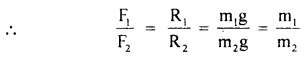4. The force of friction depends on the nature of smoothness or the state of polishing of the surfaces in contact.

Let us have a wooden plank on which blocks of wood, copper, and glass of the same mass are placed. These are pulled by a spring balance. We find that spring balance shows different readings for just starting the motion.
Hence we conclude that friction depends on the nature of the surfaces in contact.

5. The force of friction is independent of the area of the surface of contact of two bodies as long as the normal reaction is constant.

The force applied to slide the body is the same but the contact area is different for the wooden block placed on a wooden plank.

1. It helps in walking, talking, writing, sleeping, etc.
2. The brakes of vehicles can’t work without friction.
3. Moving belts remain on the rim of wheels due to friction.
4. Adhesives work due to friction.
5. Cleaning with sandpaper is due to friction.
6. Nuts and bolts are held together due to friction.

1. A significant amount of energy of a moving object is wasted in the form of heat energy to overcome friction.
2. It causes a lot of wear and tear of the parts of machinery in contact, thus reducing their lifetime.
3. It restricts the speed of moving vehicles like buses, airplanes, trains, etc.
4. The efficiency of machines decreases due to the presence of a force of friction.
5. A machine gets burnt due to the force of friction between its different moving parts due to friction.

(c) What are various methods of reducing friction?
Reduction of friction saves a lot of energy. The various methods of reducing friction are listed below:
1. Making the surfaces smooth by various methods such as:

• Polishing the surfaces and rubbing surfaces.
• Covering the surface with smooth materials such as metal foils or sun mica etc.
• Lubricating the surfaces by lubricating oils and other materials such as granite.

2. With the help of these above methods, the irregularities, grooves, etc. are filled up and the surface becomes smooth. For example in many machines grease is used to lower friction.

3. Streamlining the shape of bodies: The shape of bodies is made streamline to reduce friction. For example, the shape of high-speed buses, railway engines, ships, boats, airplanes, etc. is made of streamline to reduce fluid friction. It not only reduces friction but also helps in providing the pushing force.

4. Making changes to convert sliding into rolling friction: Since the rolling friction is much less than the sliding friction, several machine parts are designed in such a manner that they have rolling friction. For example, ball bearings are used in bicycle and machine shafts. The vehicles have wheels instead of sliding arrangements for the same reason.

Question 6.
(a) Discuss the motion of a vehicle on a level road having a circular turn.
Consider a vehicle (say a car) of mass m moving with a constant speed v on a circular level (flat) road of radius r. While taking the round, the tires of the car tend to leave the road and move away from the center of the curve. So the forces of friction f1 and f2 act inward on the two tires 1 and 2 respectively shown in fig. If R1 and R2 be the normal reactions of the ground on the tires, then
f1 = μR1 and
f2 = μR2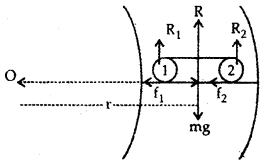If f be the total force of friction, then
f = f1 + f2
= μ(R1 + R2) = μR ….(1)

where R = R1 + R2 is the total reaction of the ground on the car.
Also R = mg (weight of the car) …. (2)

∴ From (1) and (2), we get
f = μmg …. (3)

This total- force of friction provides the necessary centripetal force $$\frac{m v^{2}}{r}$$ to the vehicle to move in the circular path.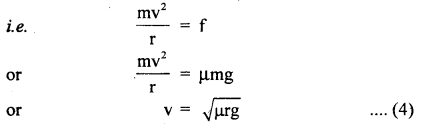Equation (4) gives the maximum speed with which the vehicle can move on the given circular road without skidding.

If its speed becomes more than the speed given by (4), then the centripetal force needed by the car will not be provided by the force of friction and hence the vehicle will skid and go off the road.

(b) What is the need for the banking of circular roads/tracks? Describe the motion of a car on a banked road.
We know that a vehicle cannot remain on a circular road if its speed is more than the speed given v = $$\sqrt{µrg}$$. In such a case, the force of friction is not sufficient to provide the necessary centripetal force. The additionally required centripetal force is obtained by banking the circular road i.e. its outer edge is raised a little above the inner edge by a suitable angle θ with the horizontal. This process is called banking and the angle is called the angle of banking.

Case I:
The motion of the car without taking into account the force of friction between the tires of car and the road:
Let OX = horizontal line
m = mass of the car
V = its velocity

The following force acts on the car:

1. Its weight mg acts vertically downwards.
2. The normal reaction of the road acts at an angle θ to the vertical.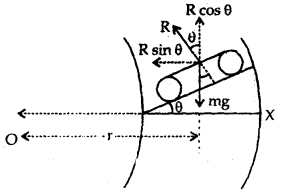Let us resolve R into two rectangular components:
R cos θ which is equal and opposite to mg
i.e. R cos θ = mg …(1)
R sin θ which acts towards the centre of the circular path provides the necessary centripetal force $$\left(\frac{\mathrm{mv}^{2}}{\mathrm{r}}\right)$$ to the car i.e.eqn. (3) gives the maximum safe speed of the car.

If it negotiates the curve on the banked road with a speed greater than that given by equation (3), then it will skid away.

Case II:
The motion of a car on a banked road taking into account the friction between the tires and the road.
The following forces act on the car:

1. Its weight mg acting vertically downwards.
2. Reaction R of the road acts at an angle θ to the verticle.
3. The force of friction between the tires and the road acts along the road inwardly.

Let us resolve f and R into rectangular components along with horizontal and vertical directions as shown in the figure.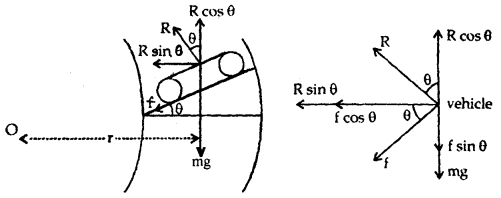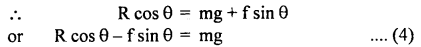Necessary centripetal force required for motion around the circular path i.e.

R sin θ + f cos θ = $$\frac{\mathrm{mv}^{2}}{\mathrm{r}}$$ ….(5)

Dividing (5) by (4), we getDividing on L.H.S. by R cos θ in numerator and denominator, we get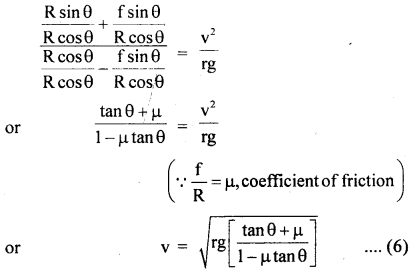Equation (6) gives the maximum speed for safely negotiating a curve on a banked road.

(c) Describe the motion of a body in a vertical circle.
When a body moves in a vertical circle, its velocity decreases from lowest point L to highest point H. Then it again increases as it moves from H to L. The.reasOn is that gravity opposes the motion of the body as it rises and helps in motion as it falls. Thus, motion is not uniform. Let m = mass of a body tied to one end of a string of length r and is rotated in a vertical circle of center O. L, H are lowest and highest points respectively.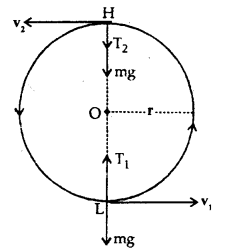Let v1 and v2 be the velocities of the body at L and H respectively which act along the tangent to the circle. mg= weight of the body which acts vertically downward both at L and H.

Also, let T1 and T2 be the tensions in the string at L and H respectively and both act towards O. Thus, the net force at L towards O is T1 – mg and provides the necessary centripetal force i.e.Similarly, T2 + mg at H provides the necessary centripetal force at H i.e.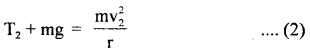Subtracting Egn. (2) from (1), we getAccording to the law of conservation of energy,
Total energy at L = Total energy at H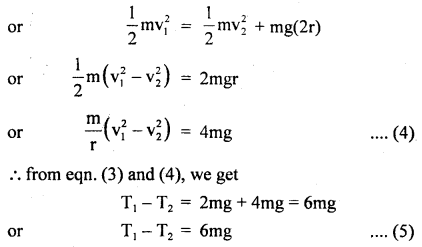i. e. in a vertical circle, the difference in tensions at the lowest and highest points is six times the weight of the body.
For completing the vertical circle successfully, the string must remain tight even at H i.e. it should not slack at H.

For this, T2 must be zero i.e. T2 = 0.

∴ From eqn. (2), mg = $$\frac{\mathrm{mv}_{2}^{2}}{\mathrm{r}}$$
or
v2 = $$\sqrt{gr}$$ ….(6)

Eqn. (6) gives the minimum velocity of the body at the highest point i.e. vmin = (rg)1/2

If v2 < $$\sqrt{gr}$$, then the string will slack at H and the vertical circle will not be completed.
From Eqn. (4) and (6), we get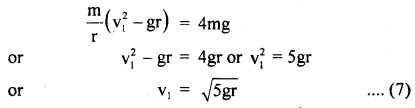Equation (7) gives the minimum velocity of projection required at the lowest point L so that the body just completes the vertical circle successfully. This phenomenon is called looping the vertical loop.

Numerical Problems:

Question 1.
A machine gun placed horizontally in front of a target fires 600 bullets per minute into the target. The average mass of the bullet is 50 gm and the bullets are fired at a speed of 800 ms-1. Calculate the average force applied to the target.
Mass of each bullet, m = 50 gm = $$\frac{1}{20}$$ kg.
v = velocity of each bullet = 800 ms-1
No. of bullet fired, n = 600,
t = 60s,
average foce exerted on the target = fav = ?
∴ p1 = initial momentum of each bullet
= mv = $$\frac{1}{20}$$ × 800 = 40 kg ms-1

∴ Total momentum of 600 bullets per min.,
Pi = nP1
= 600 × 40 = 24000 kg ms-1

As the bullets come to rest after striking the target,
∴pf = final momentum of bullets = 0

Thus change in momentum ΔP = pi – pf = 24000 kg ms
But by definition,

Impulse = average force × time
= change in momentum
or
Fav × t = Δp

∴ Fav = $$\frac{\Delta \mathrm{p}}{\mathrm{t}}=\frac{24000}{60}$$

Question 2.
A rubber ball of mass 50 gm falls from a height of 1 m and rebounds to a height of 0.5 in. Finds the impulse and average force between the ball and the ground if the time for which they are in contact is 0.1 s.
m = mass of ball = 50gm = $$\frac{50}{1000}=\frac{1}{20}$$ kg, J =?. F =?

Case I:
u = initial velocity of ball = O
h1 = height through which it falls 1m
y = final velocity of the ball =?
a = g = 9.8 ms-2
∴ using the relation,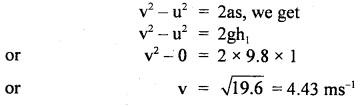If p1 be the momentum of the ball when it is about to reach the ground or before the impact,
then
p1 = mv = $$\frac{1}{20}$$ × 4.43 = 0.221 kg ms-1

Case II: u’ initial velocity = velocity after rebounding = ?
v = final velocity = 0
S = h = height up to which it rises = 0.5 m = $$\frac{1}{2}$$ m
a = – g = – 9.8 ms-2

∴ using equn. (i), we get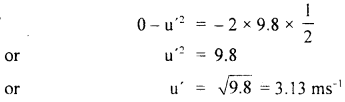If p1 = momentum of the ball after impact, then
p2 = mu = $$\frac{1}{20}$$ × 3.13 = 0.156 kg ms

∴ impulse is given byQuestion 3.
A bus moving with a velocity of 60 kmh-1 has a weight of 50 tonnes. Calculate the force required to stop it in 10s.
m = mass of bus = 50 tonnes = 50 × 1000 kg
u = initial velocity of bus = 60 kmh-1 = 60 × $$\frac{5}{18}$$ ms-1 = $$\frac{50}{3}$$ ms-1
v = final velocity of bus = 0
t = 10 s, a = ? and retarding force, F = ?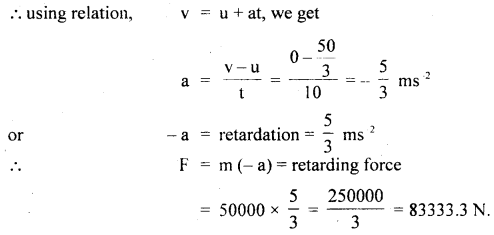Question 4.
A hunter has a machine gun that can fire 50 g bullets with a velocity of 150 ms-1. A 60 kg tiger springs at him with a velocity of 10 ms-1. How many bullets must the hunter fire into the tiger so as to stop him in his track?
m = mass of bullet = 50 gm = 0.050 kg
M = mass of tiger = 60 kg
v = velocity of bullet = 150 ms-1
V = velocity of tiger = – 10 ms-1
(∵ it is coming from opposite direction) .

Let n = no. of bullets fired per second at the tiger so as to stop it.
∴ pi = 0 before firing …. (i)
pf = n(mv) + MV …. (ii)

∴ According to the law of conservation of momentum,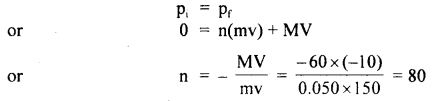Question 5.
A mass of 200 kg rests on a rough inclined plane of angle 300. If the coefficient of limiting friction is $$\frac{1}{\sqrt{3}}$$ find the greatest and the least forces in newton, acting parallel to the plane to keep the mass ¡n equilibrium.
Here, m = mass of body = 200 kg
Let angle of inclination = θ
μs = coefficient of limiting friction = $$\frac{1}{\sqrt{3}}$$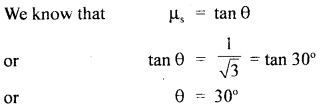The rectangular components of mg are as shown in fig.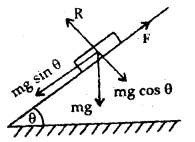Here mg sin θ acts along the plane in the downward direction and is given by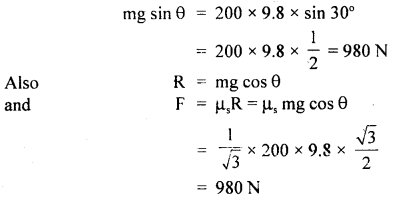(i) the least forces in newton, acting parallel to the plane to keep the mass in equilibrium. given by
f21 =mg sinθ – F = 980 – 9800 = 0

(ii) The greatest force to be applied to keep the mass in equilibrium is given by
f2 = mg sin θ + F = 980 + 980 = 1960 N.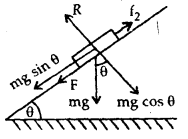Question 6.
Find the force required to move a train of 2000 quintals up an incline of 1 in 50, with an acceleration of 2 ms-2, the force of friction being 0.5 newtons per quintal.
Here, m = 2000 quintals
= 2000 × 100 kg (v 1 quintal = 100 kg)
sin θ = $$\frac{1}{50}$$ , acceleration, a = 2 ms-2

F = force of friction
= 0.5 N per quintal
= 0.5 × 2000 = 1000 N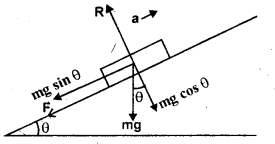In moving up an inclined plane, force required against gravity
= mg sin 0
= 2000 × 100 × 9.8 × $$\frac{1}{50}$$
= 39200N.
Also if f = force required to produce acce. = 2 ms-2.
Then f = ma = 200000 × 2 = 400000 N

∴Total force required = F + mg sin θ + f
= 1000 + 39200 + 400000 = 440200 N.

Question 7.
A bullet of mass 0.01 kg is fired horizontally into a 4 kg wooden block at rest on a horizontal surface. The coefficient of kinetic friction between the block and the surface is 0.25. The bullet remains embedded in the block and the combination moves 20 m before coming to rest. With what speed did the bullet strike the block?
Here, m1 = mass of the bullet = 0.01 kg
m2 = mass of the wooden block = 4 kg
μ2 = coefficient of kinetic friction = 0.25
initial velocity ofblock, u2 = 0, s = distance moved by combination=20 m

Let u1 = initial velocity of the bullet
If v = velocity of the combination, then according to the principle of conservation of linear momentum,If F = kinetic force of friction,
ThenThen retardation ‘a’ produced is given by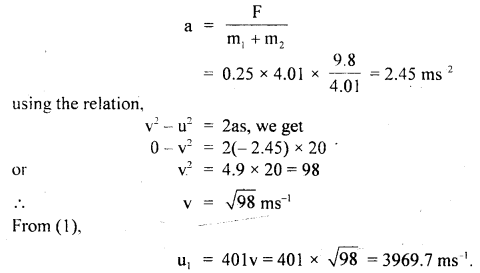Question 8.
A force of 100 N gives a mass m1 an acceleration of 10 ms-2, and of 20 ms-2 to a mass m2. What acceleration would it give if both the masses are tied together?
Let a = acceleration produced if m1 and m2 are tied together.
F = 100 N, Let a1 and a2 be the acceleration produced in m1 and m2 respectively.
∴ a1 = 10 ms-2, a2 = 20 ms-2 (given)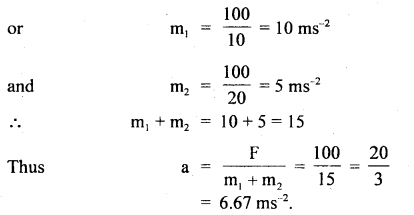Question 9.
A balloon with mass M is descending down with an acceleration ‘a’ < g. What mass m of its contents must be removed so that it starts moving up with an acceleration ‘a’?
Let F = retarding force acting on the balloon in the vertically upward direction.When the balloon is descending down with an acceleration ‘a’, then the net force acting on the balloon in the downward direction is given
by
Ma = Mg – F
or
F = Mg – Ma ….(i)
When the mass m is taken out of the balloon, then its weight is
= (M – m)g

Now as the balloon is moving upward with acceleration ‘a’, so the net force acting on the balloon in the upward direction is given by: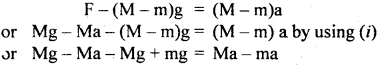Question 10.
Three blocks are connected as shown below and are on a horizontal frictionless table. They are pulled to right with a force F = 50 N. If m1 = 5 kg, m2 = 10 kg and m3 = 15 kg, find tensions T3 and T2.
Here, F = 50 N, m1 = 5 kg, m2 = 10 kg m3 = 15 kg.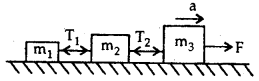As the three blocks move with an acceleration ‘a’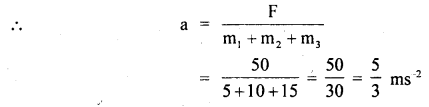To determine T2: Consider the free body diagram (1). Here F and T2 act towards the right and left respectively.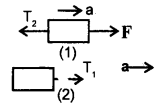As the motion is towards the right side, so according to Newton’s Second law of motion: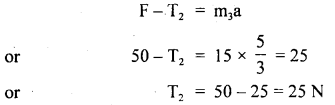To determine T3: Consider the free body diagram (2)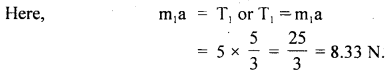Question 11.
A monkey is descending from the branch of a tree with constant acceleration. If the breaking strength is 75% of the weight of the monkey, then find the maximum acceleration with which the monkey can slide down without breaking the branch.
Let a = constant acceleration of the monkey descending from the branch of tree = T∴ According to Newton’s Second law of motion,∴ from (1) and (2), we getQuestion 12.
A body of mass 1 kg initially at rest explodes and breaks into three fragments of masses in the ratio 1:1:3. The two pieces of equal mass fly off perpendicular to each other with a speed of 30 ms-1 each. What is the velocity of the heavier fragment?
Let m1, m2, and m3 be the masses of the three fragments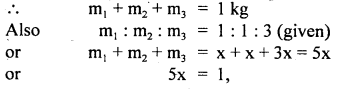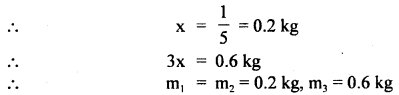Also let v and v2 be the velocities of the two fragments. ..
∴ v1 = v2 = 30 ms-1
If v3 be the velocity of the heaviest fragment, then according to the law of conservation of linear momentum in the horizontal direction,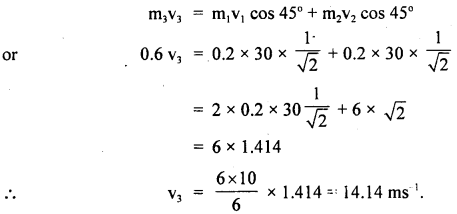Question 13.
A mass of 4 kg is suspended by a rope of length 30 m from the ceiling. A force of 30 N in the horizontal direction is applied at the mid-point of the rope. What is the angle, the rope makes with the vertical in equilibrium? Take g = 10 ms-2 and neglect the mass of the rope.
OA = OB 1.5 m
Let ∠AOC = 0
F = horizontal force = 30 N
m = mass of the body = 4 kg
mg = 4 × 10 = 40 N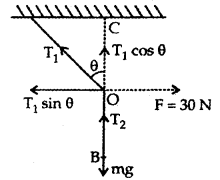Let T1 and T2 be the tensions in parts OA and OB respectively, where O = midpoint of the rope
∴ T2 = mg = 40 N
At point O, three forces T2, T1, and 30 N are acting.

Let us resolve T1 into rectangular components.
Horizontal component of T1 = T1 sin θ
Vertical component of T1 = T1 cos θ
Now T1 cos θ =40 ….(i)
and T1 sin θ = 30 …. (ii)

Dividinig (ii) by (i), we get

tan θ = $$\frac{3}{4}$$ = 0.75 = tan 36.87°
θ = 36.87°.

Question 14.
An elevator weighs 4000 kg. when the upward tension in the supporting cable is 48000 N, what is the upward acceleration? Starting from rest how far does it rise in 3s?
Weight of elevator,
W = Mg = 4000 kgf = 4000 × 9.8 N
= 39200 N
mass of elevator, M = 4000 kg

F = Force on elevator in upward direction = upward tension in the cable = 48000 N.
If F’ = Net force on the elevator in the upward direction
Then F’ = F – W = 48000 – 39200 = 8800 N

If a = upward acceleration,
Then a = $$\frac{\mathrm{F}^{1}}{\mathrm{M}}=\frac{8800}{4000}=\frac{11}{5}$$ = 2.2 ms-2

Let S be the distance covered by the elevator in 3s. For upward motion, we have
u = 0,a = 2.2ms-2, t = 3s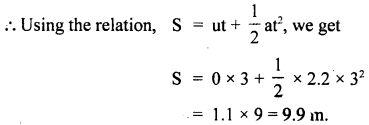Question 15.
A disc of mass 10 gm is kept floating horizontally by throwing 10 marbles per second against-it from below. If the mass of each marble is 5 gm, calculate the velocity with which the marbles are striking the disc. Assume that the marbles strike the disc normally and rebound downward with the same speed.
When each marble hits the disc, it produces an impulsive force and acts against the weight of the disc. This force keeps the disc floating for $$\frac{1}{10}$$ s.

Let v = velocity with which each marble strikes the disc and rebounds downward.
Impulse imparted by each marble = charge in momentum
= mv – (- mv) = 2 mv

Since m = 5g = 5 × 10-3 kg
∴ Impulse = 2 × 5 × 10-3v
= 10-2 v kg ms-1.

Now average force between the disc and each marble = weight of the disc.
The resultant downward force produces the change in momentum.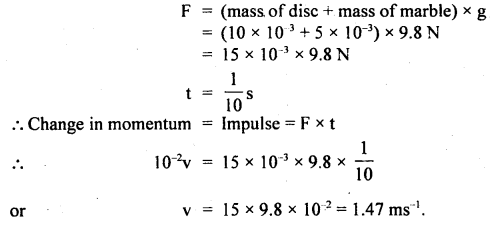Question 16.
A box is placed on a horizontal measuring scale that reads zero when the box is empty. A stream of pebbles is then dropped in to the box from a height h above its bottom at the rate of n pebbles per second. Each pebble has a mass m. If the pebbles collide with the box such that they immediately come to rest after collision. Find the scale reading at time t after the bubble begins to fill the box.
Let v = speed the pebble when it strikes the box.
Then using v2 – u2 = 2as, we get
v2 – 0 = 2gh (∵ here a = g, S = h, u = 0)
or
v = $$\sqrt{2gh}$$

Mass of each pebble = m
Momentum of each pebble = mv = m $$\sqrt{2gh}$$
Each pebble comes to rest after colliding the box

∴ Change in momentum during each collision = m $$\sqrt{2gh}$$
Δt = Time for each colbsion = $$\frac{1}{n}$$s

Force exerted by pebbles on the box,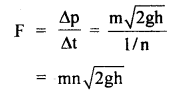Weight of pebbles after time t is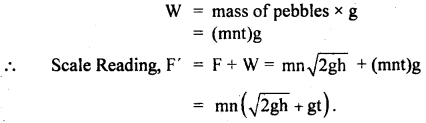Question 17.
A stone of mass 5 kg falls from the top of a cliff 40 m high and buries itself 2 m deep in the sand. Find the average resistance offered by the sand and the time it takes to penetrate (g = 9.8 ms-2).
Let the acceleration of the stone in the sand be “a”.
The velocity it gains by falling through a height of 40 m is
v = $$\sqrt{2gh}$$ = $$\sqrt{2 \times 9.8 \mathrm{~ms}^{-2} \times 40 \mathrm{~m}}$$
= 28 ms-1 = u = initial velocity

When it buries in the sand its final velocity is zero so the acceleration
a = $$\frac{0^{2}-\left(28 \mathrm{~ms}^{-1}\right)^{2}}{2 \times 2 \mathrm{~m}}$$ = -196 ms-1

(i) The average resistance offered by the sand is
F = ma = 5 kg × 196 ms-2 = 980 N

(ii) Let t be the time of penetration in the sand
using equation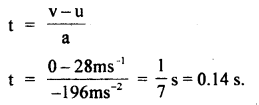Question 18.
An airplane required a speed of 80 km h-1 for takeoff on a 100 m long runway. The coefficient of friction between the tires of the plane and the runway is 0.2. Assuming that the plane accelerates uniformly, what is the force required by the engine to take off the plane? The mass of the airplane is 10,000 kg.
Take off speed of plane, v = 80 km h-1
Run-on the ground, s = 100 m
u = initial velocity = 0
Acceleration ‘a’ of the plane is obtained from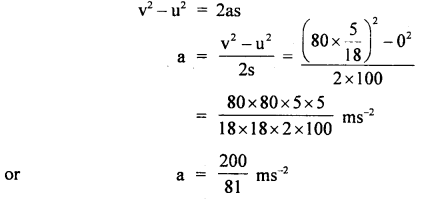The force to produce this acceleration = ma= 10,000 × $$\frac{200}{81}$$ N
Force needed to overcome friction = μm = μmg
= 0.2 × 10,000 × 9.8 N

The force required to be given to the engine is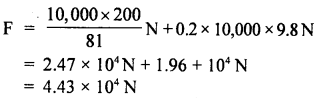Question 19.
A 4 m long ladder of aluminum having 20 kg mass rests against a smooth wall making an angle of 30° with it. Find the coefficient of friction between the ladder and the ground so that ladder does not slip. Take g = 10 ms4.
Let the ladder AB of length 4 m rest against smooth wall BC. The reaction of the ground is say R1 and that of the wall on the ladder is R2. The weight of the ladder acts at the midpoint O in a vertically downward direction (assuming the ladder to be uniform).

Let F be the force of friction.
Taking moments of mg and R2 about A, we get
mg × AD = R2 × BC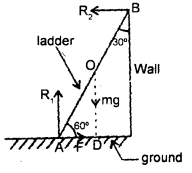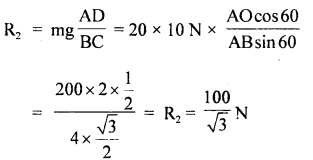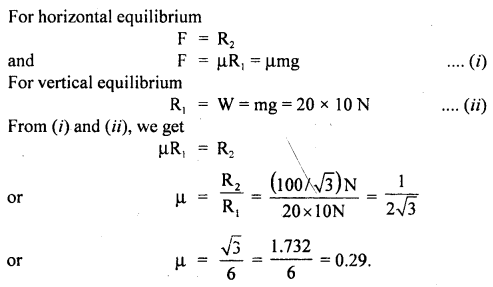Question 20.
Prove that a motor car moving over a convex bridge is lighter than the same car resting on the same bridge.
The motion of the motor car over the convex bridge is the motion along the segment of a circle. The centripetal force is provided by the difference of weight mg of the car and the normal reaction R of the bridge.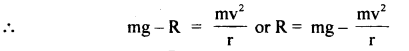∴R = apparent weight of the moving car
Clearly R < mg i.e. the weight of the moving car is less than the weight of the stationary car.Question 21.
A hammer weighing 1 kg moving with a speed of 10 ms-1 strikes the head of a nail driving it 10 cm into a wall. Neglecting the mass of the nail, calculate:
(a) the acceleration during the impact.
(b) the time interval during the impact,
(c) the impulse.
Here, the initial velocity of nail = velocity of the hammer
i. e. u = 10 ms-1
Final velocity of nail, v = 0, m = mass of hammer = 1 kg
Distance covered, s = 10 cm = 0.1 m

Let t be the time interval for which impact lasts and let a be the acceleration produced =?
(а) Using the relation,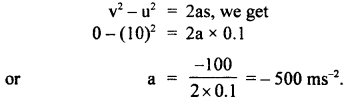(b) Also using the relation,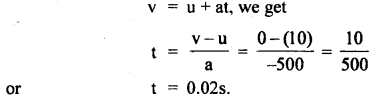(c) Impulse
Ft = (ma)t
= 1 × (-500) × 0.02
= – 10 Ns.

Question 22.
A rocket with a lift of mass 20,000 kg is blasted upward with an initial acceleration of 5 ms2. Calculate internal thrust of the blast
Here, m = 20,000 kg
a = 5 ms-1
∴ F = ma = 20,000 × 5N = 105 N

Let T = upward of thrust and
W = weight of rocket.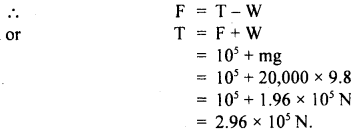Question 23.
A car starts from rest and accelerates uniformly with 2ms2. At t = 10 s, a stone is dropped out of the window (1m high) of the car. What are the : (a)velocity, (b) acceleration of the stone at t = 10.ls. Neglect air resistance and take g = 9.8 ms2.
(a) Here, the stone will possess horizontal velocity due to the motion of the car and vertical downward velocity due to gravity.
Here u = 0, a = 2 ms-2, t = 10s, g = 9.8 ms-2

If vH and vy be its horiìontal and vertical velocities respectively, then using relation,
v = u + at, we get
vH = u + at = 0 + 2 × 10 = 20 ms-1
and
vy = u + gt = 0 + 9.8 × 0.1 = 0.98 ms-1

If $$\overrightarrow{\mathbf{v}}$$ be its resultant velocity at < θ with horizontal direction, then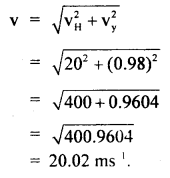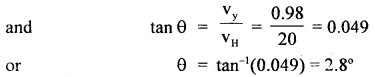The vertical component of acceleration,
g = 9.8 ms-2

Since the velocity is constant as no force acts on the stone at the time of dropping, so the horizontal component of acce. is zero.

If a’ be its acce. at 10.1 s, then
a’ = $$\sqrt{\mathrm{g}^{2}+0^{2}}$$ = g = 9.8 ms-2.

Question 24.
A stream of water flowing horizontally with a speed of 15 ms-1 gushes out of a tube of cross-sectional area 10-2 m2 and hits at a vertical wall nearby. What is the force applied on the wall by the impact of water, assuming it does not rebound? The density of water is 1000 kg m-3.
Here, A = area of cross-section of tube
= 10-2 m2
v = speed of water = 15 ms-1
ρ = density of water = 103 kg m-3

If V be the volume of water striking the wall per second, then
V = Av = 10-2 × 15 = 0.15 m3 s-1

Let m be the mass of water striking/sec.
∴ m = Vρ = 0.15 × 1000 = 150 kg s-1

Let pi = initial momentum of water striking the wall s-1,
∴ Pi = mv
= 150 × 15 = 2250 kg ms-2

pf = final momentum of water/sec = 0 as it does not rebound
∴ Δp = change in momentum/s = pf – Pi
= 0 – 2250 = – 2250 kg ms-2
or
F = 2250 N.

Question 25.
A body of mass 1 kg lies on a rough horizontal plane. A horizontal force of 15 N produces in the body an acceleration of 10 ms”2. Find the force of friction and coefficient of friction between the body and the table.
Here, m = mass of body = 1 kg
F = force = 15 N
a = acceleration = 10 ms-2

Let f be the force of friction and μ, be the coefficient of friction
∴ the net force on the body = F – fValue-Based Type:

Question 1.
Anil is a student of the science stream who lived on the first floor of a building. One day he and his grandfather were enjoying the holiday. Suddenly he observed that dense smokes are coming from his neighbor’s flat. People were crying and panic was there. There was a rush on the staircase. He was thinking about how he could save his grandfather. Suddenly an idea came to his mind. He took a turban cloth and suspended it on the ground and asked a person to hold the other end of the cloth tightly; Then he asked his grandfather to gently sit on the turban cloth. He was now able to comfortably reach the ground:
(i) What values and qualities displayed by Anil?
The values displayed by Anil are Caring, Presence of mind, Courageous, and affection to elderly people.

(ii) Which concept is being used here?
Motion on a Rough inclined plane

(iii) What will be the tension in the cloth inclined at an angle of 30° from horizontal when a person of mass 60 kg falls through it with an acceleration Of 2 m/s2Here, m = 60 kg, θ = 30°, a = 2m/s2, g = 10 m/s2
∴ Tension T =mg sin θ – ma
= m(g sin θ – a)
= 60(10 × sin30°- 2)
= 60 × (10 × $$\frac{1}{2}$$ – 2)
= 60 × 3 = 180N

Question 2.
Two friends Vipul and Mohan are cycling at 18 km/h on a level road. Vipul told that there is a sharp circular turn of the radius of 3 m. So, reduce your speed to avoid slipping. But Mohan was a careless boy and he did not follow his advice. Suddenly he slipped and felt the importance of his friend’s idea.
(i) Which values are depicted by Vipul?
Cooperative, caring, awareness, and helping nature.

(ii) Explain why the cyclist Mohan slip while taking the turn?
[Take us = 0.1 ]
If the speed is too large or if the turn is too sharp (i.e. of too small a radius) or both, the frictional force is not sufficient to provide the necessary centripetal force, and the cyclist slips. The condition for the cyclist not to slip is given by: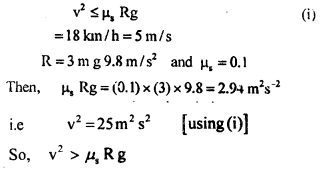Hence, the condition is not obeyed. So, the cyclist will slip while taking the circular turn.

Question 3.
Mahesh was driving a car with his old grandfather. After some time when the destination was about to come, he did not apply the brakes. But he stopped the engine. Even then the car was running on the road for some time. His grandfather surprised and asked his grandson the reason for the car running without the engine on. Mahesh was a student of the science stream of class XI. He explained that it is due to the momentum of the car.
(i) Which values are displayed by Mahesh here?
The values displayed by Mahesh are:
Intelligence, awareness, helping nature, and willingness to explain the scientific reason, (i.e explanation).

(ii) What is momentum and on which factors it depends?
The momentum of a body is defined as the product of its mass and velocity.
i.e Momentum = mass × velocity
or
P = m × v
Momentum is directly proportional to mass as well as its velocity.
So, Momentum depends on the mass and the velocity of a moving body.

Question 4.
Suresh noticed a big Granite Rock in his locality. He thought that if they worked upon it they could earn money. He took permission from the Government, completed all the formalities. He broke the Rock using a bomb. The rock was made into slices. They established a Granite industry. Many of the people in the surroundings started to earn and live comfortably.
(a) What values of Suresh impress you?
Suresh knows how to utilize the natural resources, has got concerned for others. Also, he knows how to complete all legal formalities before taking up any work.

(b) A bomb is thrown in a horizontal direction with a velocity of 50 m/s. It explodes into two parts of masses 6 kg and 3 kg. the heavier fragment continues to move in the hori¬zontal direction with a velocity of 80 m/s. Calculate the velocity of the lighter fragment.
Solution: r
According to low of conservation of momentum
Total momentum of fragments = Momentum of the Bob
m1 v1 + m2 v2 = M V
⇒ 6 × 80 + 3 × V2 = 9 × 50
⇒ V2 = -10 m/s.

Question 5.
Rakesh with an intention to win in the interschool sports practiced the high jump every day for about a month. He participated and won I position in the interschool sports.
(a) Comment upon the values Rakesh possesses.